# Set

Jump to: navigation, search

A set is a collection of objects called elements. Sets are the subject of set theory. Two sets are the same if they contain the same elements, regardless of the order they are in. Sets are written in set notation, such as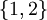indicating the set containing the elements 1 and 2. This is the same as the set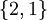because order does not matter when comparing sets.

Also, repetition of elements is irrelevant, so the setis the same as the set.

An important concept in set theory is cardinality. In the case of finite sets, this is simply the number of elements of the set. Sohas a cardinality of 2. However, the theory is more complicated in the case of infinite sets.

It was thought that an informal concept of sets (Naive set theory) was sufficient, however Russell's Paradox shows that this can lead to a contradiction if sets are allowed to contain themselves. Modern set theory is more formal, and disallows such paradoxical sets.

## Examples

Let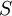be the set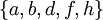, and let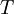be the set• The cardinality ofis.
• The cardinality ofis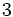.
• The intersection,is the set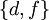. This is because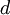and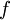are the only two elements in bothand.
• The union,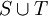is the set. These six elements are in the union because they belong to, to, or to bothand.
• The set-theoretic difference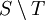(alternative notation: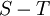) is the set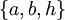containing all the elements inthat are not in.
• The power set of,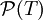, consists of all subsets ofincluding the empty set anditself. One can check that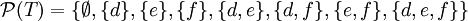. Exercise: How many elements are in?

Let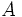be the set of all prime numbers, and letbe the set of all even numbers. What is the intersection,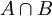? It is a set containing one element, 2. Since any even number greater than 2 has two as a proper factor, no even number aside from 2 belongs to. However, 2 is a prime number. Thus, the intersection is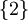.

Let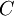be the set of all positive integers that are multiples of, and let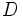be the set of all positive integers that have a remainder of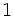when divided by. The intersection,, is the empty set, because no positive integer that is a multiple ofwill have remainderwhen divided by. However, if we taketo be the set of even numbers, as before, thenis not empty. Exercise: What are the elements of?

## Examples of sets in the real world

There is the set of unborn children who were aborted, about which striking conclusions can be drawn. Given the large and diverse number of elements of this set, it would likely include many who could surpass existing athletic and intellectual achievements. Indeed, many of the world records and Nobel Prize achievements recognized today would have been outdone by members of this set.

Another striking example is the how traditional marriage provides a greater set than otherwise: the union of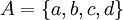and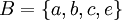is merely, while the union of a man,and a woman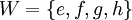, is, which is a broader and more diverse set.

Define "A" as the set of all false assertions, and "B" as the set of all the Bible passages. Since there are no Counterexamples to the Bible, the intersection between set A and set B is the empty set. On the other hand, if "C" is set of evolutionary assertions, then the intersection between set A and set C is not empty because it contains Counterexamples to Evolution.

## Application -- Combinatorics

Sets, and their cardinalities, are used in some very important aspects of combinatorics, that is, the study of combinations. Letand.

The cardinalities of both are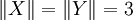.

Let. The vertical bar means "such that", and the epsilon-like symbol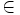means "is an element of". So this means that S is the collection of sets which are pairs x and y such that x is in X and y is in Y.

We can enumerate this..

Its cardinality is.

Now try a harder example, based on the same two sets. Let.

Now, so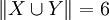.

The problem of finding the number of subsets of a set that has some particular cardinality is the combination choosing problem, and shows up in many areas of probability and statistics. In this case, it's the problem of choosing 2 elements out of a set of 6 elements. The number of such choices is often written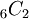or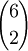, and is often pronounced "six choose two". These numbers are also called the binomial coefficients because they appear as coefficients in the expansion of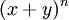. In this case,is the coefficient of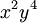in.

There is a simple formula for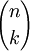. It is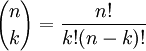.

In the case of the set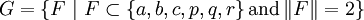, the set is.

Its cardinality is 15.

We can check that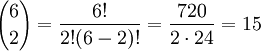.